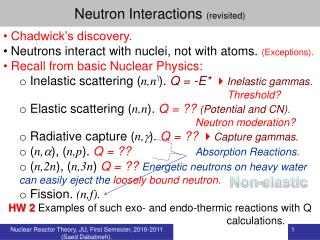DownloadDownload PresentationNeutron Interactions (revisited)

# Neutron Interactions (revisited)

Download Presentation## Neutron Interactions (revisited)

- - - - - - - - - - - - - - - - - - - - - - - - - - - E N D - - - - - - - - - - - - - - - - - - - - - - - - - - -
##### Presentation Transcript

1. Neutron Interactions (revisited) • Chadwick’s discovery. • Neutrons interact with nuclei, not with atoms. (Exceptions). • Recall from basic Nuclear Physics: • Inelastic scattering (n,n\). Q = -E* Inelastic gammas. Threshold? • Elastic scattering (n,n). Q = ?? (Potential and CN). Neutron moderation? • Radiative capture (n,). Q = ?? Capture gammas. • (n,), (n,p). Q = ?? Absorption Reactions. • (n,2n), (n,3n) Q = ?? Energetic neutrons on heavy water can easily eject the loosely bound neutron. • Fission. (n,f). • HW 2 Examples of such exo- and endo-thermic reactions with Q calculations. Non-elastic Nuclear Reactor Theory, JU, First Semester, 2010-2011 (Saed Dababneh).

2. Neutron Scattering (revisited) • Elastic or inelastic. • Analogous to diffraction. • Alternating maxima and minima. • First maximum at • Minimum not at zero (sharp edge of the nucleus??) • Clear for neutrons. • Protons? High energy, large angles. Why? • Inelastic  Excited states, energy, X-section and spin-parity. Nuclear Reactor Theory, JU, First Semester, 2010-2011 (Saed Dababneh).

3. Reaction Cross Section (revisited) • Probability. • Projectile a will more probably hit target X if area is larger. • Classically:  = (Ra + RX)2. • Classical  = ??? (in b)n+ 1H, n + 238U, 238U + 238U • Quantum mechanically:  =  2. • Coulomb and centrifugal barriers  energy dependence of . • What about neutrons? • Nature of force: • Strong: 15N(p,)12C  ~ 0.5 b at Ep = 2 MeV. • Electromagnetic: 3He(,)7Be  ~ 10-6 b at E = 2 MeV. • Weak: p(p,e+)D  ~ 10-20 b at Ep = 2 MeV. • Experimental challenges to measure low X-sections.. Nuclear Reactor Theory, JU, First Semester, 2010-2011 (Saed Dababneh).

4. Reaction Cross Section (Simple terms) A (Area of what??!!) |v| X Position of a neutron 1 s before arriving at target Target with N atoms.cm-3 or NAX atoms. Monoenergetic (and unidirectional) neutrons of speed v (cm.s-1) and density n (cm-3) NX?? Volume = vA containing nvA neutrons that hit the “whole!!” target in 1 s. Beam Intensity InvA/A = nv (cm-2s-1) Number of neutrons interacting with target per second  I, A, X and N = t I N A X Total microscopic cross section Nuclear Reactor Theory, JU, First Semester, 2010-2011 (Saed Dababneh).

5. Reaction Cross Section (Simple terms) • Number of neutrons interacting with target per second • = t I N A X • Number of interactions with a single nucleus per second • = t I Interpretation and units of . • nvA= IA neutrons strike the target per second, of these • tI neutrons interact with any single nucleus. Thus, • measures the probability for a neutron to hit a nucleus (per unit area of target). Study examples in Lamarsh Total microscopic cross section Total number of nuclei in the target Effective cross-sectional area of the nucleus. Nuclear Reactor Theory, JU, First Semester, 2010-2011 (Saed Dababneh).

6. Reaction Cross Section (Simple terms) The probability for a neutron to hit a nucleus (per unit area of target): Function of what? Typical nucleus (R=6 fm): geometrical R2  1 b. Typical : <b to >106 b. Nuclear Reactor Theory, JU, First Semester, 2010-2011 (Saed Dababneh).

7. Reaction Cross Section (Simple terms) Number of neutrons interacting with target per second = t I N A X Number of interactions per cm3 per second (Collision Density) Ft= t I N = I t t= N t Study examples in Lamarsh Total microscopic cross section Volume of the target Macroscopic total cross section. Probability per unit path length. Attenuation not moderation ! Mean free path Nuclear Reactor Theory, JU, First Semester, 2010-2011 (Saed Dababneh).

8. Neutron Attenuation X I0 I Recall t= N t • mfp for scattering ls = 1/Ss • mfp for absorption la= 1/Sa • …………. • total mfp lt = 1/St Probability per unit path length. Probability Nuclear Reactor Theory, JU, First Semester, 2010-2011 (Saed Dababneh).

9. Reaction Cross Section (Simple terms) Homogeneous Mixture Molecule xmyn Nx=mN, Ny=nN given that events at x and y are independent. Study examples in Lamarsh Nuclear Reactor Theory, JU, First Semester, 2010-2011 (Saed Dababneh).

10. Reaction Cross Section Look for b Detector for particle “b” d Ia\ “b” particles / s , cm2 “X“ target Nuclei / cm2 “a” particles / s Nuclear Reactor Theory, JU, First Semester, 2010-2011 (Saed Dababneh).

11. Reaction Cross Section Many different quantities are called “cross section”. Krane Table 11.1 Angular distribution Units … ! “Differential” cross section (,) or ( ) or “cross section” …!! Doubly differential t for all “b” particles. Nuclear Reactor Theory, JU, First Semester, 2010-2011 (Saed Dababneh).

12. Nuclear Reactor Theory, JU, First Semester, 2010-2011 (Saed Dababneh).

13. n-TOF CERN Nuclear Reactor Theory, JU, First Semester, 2010-2011 (Saed Dababneh).

14. Nuclear Reactor Theory, JU, First Semester, 2010-2011 (Saed Dababneh).

15. Neutron Cross Section (Different Features) 1/v Fast neutrons should be moderated. 235U thermal cross sections fission  584 b. scattering  9 b. radiative capture  97 b. Fission Barriers Nuclear Reactor Theory, JU, First Semester, 2010-2011 (Saed Dababneh).